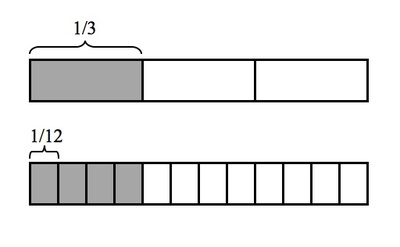Painting a room

Alignments to Content Standards: 5.NF.B.7.a

Kulani is painting his room. He needs $\frac13$ of a gallon to paint the whole room. What fraction of a gallon will he need for each of his 4 walls if he uses the same amount of paint on each? Explain your work and draw a picture to support your reasoning.

IM Commentary

The purpose of this task is to provide students with a situation in which it is natural for them to divide a unit fraction by a non-zero whole number. Determining the amount of paint that Kulani needs for each wall illustrates an understanding of the meaning of dividing a unit fraction by a non-zero whole number. Students might first attempt to draw Kulani's room in order to solve this problem instead of drawing the strip diagram that is given in the solution below. It is important to motivate students to draw the a picture that represents the amount of paint because it is the $\frac{1}{3}$ of the gallon of paint that is being divided into 4 pieces rather than the entire room being divided by the amount of paint.

Solution

In order to find out what fraction of a gallon of paint Kulani will use on each wall, we need to divide the amount of paint that he has, $\frac{1}{3}$ of a gallon, by 4.

We know that the fraction $\frac{1}{3}$ represents 1 piece where 3 equal pieces make a whole gallon. We can draw a rectangle that represents a whole gallon and shade $\frac13$ of it to represent the amount of paint he has. If we divide each of the 3 equal pieces in the picture into 4 smaller, equal-sized pieces, then the whole gallon is now divided into $4\times3=12$ equal pieces. This means that one of these new, smaller equal pieces represents the fraction $\frac{1}{12}$:Note that by dividing every third into 4 equal pieces, we divided the $\frac{1}{3}$ that represents Kulani's paint into 4 equal pieces, and in particular each of these pieces is $\frac{1}{12}$ of the whole gallon. This means that $\frac{1}{3}\div4=\frac{1}{12}$. Based on our understanding of the relationship between multiplication and division, we can also see that $4\times \frac{1}{12}=\frac{1}{3}$.

So Kulani will need to use $\frac{1}{12}$ of a gallon of paint on each wall.